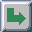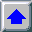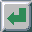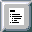Next: CRTs Up: Display Devices Previous: Display Devices

# Slides and Goldberg Rule

An image is created on a slide as the result of a chemical process. The light arriving on the film through the lenses causes some chemical reactions on the emulsion that is on the film, and an image is formed. The film is then processed, and eventually processed once more to obtain photographic prints.

Various films are often described using an ``H-D'' plot (H-D stands for Hurter and Driffield who devised them in 1890 [JaMe66]). An H-D plot describes a density as a response to a given exposure. Let us define exposure and density next.

Exposure is defined as the product of the irradiance incident upon the photosensitive surface (I) and the time during which the surface is exposed (t):Photographic exposure is usually given in photometric rather than radiometric units. In equation 3.1, I is given inor lux and exposure is then in lux-sec. In the cases where the incoming flux is a continuous spectral distribution, the exposure is given by the integral:The measured response of a photographic material is given in density. Density is a unitless, logarithmic measure that indicates the opacity of an emulsion that results from processing.

Transmission density is used for describing the response of photographic film. It is defined as:where T is a transmittance value,, that gives the ratio of light transmitted through an emulsion to the quantity of light incident to it. There is also the reflective densitywhich is used in describing the response of photographic papers.

Let us consider now, a color slide with characteristic curves as in fig. 3.3. The horizontal axis shows the logarithm of the exposure, the vertical axis shows the densities in the r,g,b channels.

The density ranges of the three color channels are somewhat different from another, hence an achromatic gray can only be produced in the coincident part of the density ranges. It can be seen in fig. 3.3 that the density ranges exceed 3. In linear terms: the contrast exceeds 1000! The corresponding illumination range on the horizontal axis is [-2.5, -0.1]. Values less then -2.5 and greater then -0.1 cause no change in densities (so called ``fog'' and ``flare'' regions). That means, roughly, that the logarithm of the range of exposition is 2.4, a value of about 250 on a linear scale! This is much higher than the displayable effective contrast on any other medium. Dynamic ranges of black and white glossy photo papers can be up to 60, but for all other media including color photos and prints it is significantly smaller, in a range of 10 to 40. In photography, only the straight curve sections are convenient. Moreover, the standard is a paper print made from the slide, perceptionally correct for a contrast rendering of 1:1. This means that we want to reproduce the original contrast of 50 as 50 on the final print. Therefore, the really applicable range of contrast for exposures does not exceed the value of 45, that is about 1.65 in(actually the recommended contrast value in photography is 32). For instance, on the horizontal axis of fig. 3.3 the section [-0.25,-1.9] may be applied. This corresponds to the density range [0.2, 2.7] on the vertical axis. That means that the original scene contrast ofis mapped to a contrast ofon the slide.Figure 3.3: The characteristic curves of Kodak Ektachrome Panther 100x Professional

Slide gamma is defined as the slope of the straight part of the characteristic seen in fig. 3.3. Slide gamma values vary from 1.5 to 1.7, and this is the reason why the scene contrast is enlarged on the slide. The question emerges why the gamma value is not 1. Obviously, on a paper print (enlargement) gamma must be 1, otherwise the print would not be equivalent to the original appearance. Remember that we want to reproduce original contrast exactly. This is possible since photo papers have gamma values as well. Enlargements from slides are made on so called positive papers, with gamma values less than 1. The resultant gamma is the product of the gammas of the slide and of the positive paper, in fact, about 1. Actually the original scene contrast will be enlarged on slide, and then again decreased by the use of a positive photo paper. Similarly, soft color negatives, with gamma values less than 1, and enlargement papers of hard gradation (with gamma values over 1) have resultant gamma values of about 1. The original contrast is changed on slides due to avalue of 1.5 to 1.7. Even the color components are changed from r, g, b to,,. The question emerges how it is possible that slides are, despite all these facts, perceived correctly when projected. A projected slide enlarges the original contrast, but the perceived image looks correct. The Goldberg rule gives us the answer [Schr81]. The Goldberg rule states that the way we see something depends in an exponential way (Goldberg-Gamma) on the surrounding lighting. If the human eye is adapted to a very dark surrounding, a Goldberg-Gamma value of 1.5 to 1.7 gives perceptually correct results. For eyes adapted to brightness the Goldberg-Gamma decreases down to 1. That is one of the reasons why slides viewed in a well lit room do not look satisfactory, but when they are projected in a dark room they are just perfect. In other words, our visual system reduces the contrast in the dark surrounding, and high contrast projection is perceived as normal contrast image. On the other hand if a slide is viewed in a bright surrounding, its contrast is too high, and it looks somehow contrast-less. This paradox that high contrast image seems to have low contrast, and a low contrast image looks as full of contrast will be described in the section 4.2.

Note, that it would be possible to exploit slide characteristic in another way. We could compute a raw image, and compress the original contrast of let's say 300 to 50, and use it as input to the film writer. The resulting slide will then increase input contrast to the original 300 value. In this case the slide should be viewed in a well lit room, placed on a diffuse light source. If there were a big enough slide, it would outperform all common display media this way.Next: CRTs Up: Display Devices Previous: Display Devices

matkovic@cg.tuwien.ac.at